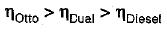Courses

# Air Standard Cycle Of IC Engine - 2

## 10 Questions MCQ Test Mock Test Series - Mechanical Engineering (ME) for GATE 2020 | Air Standard Cycle Of IC Engine - 2

Description
This mock test of Air Standard Cycle Of IC Engine - 2 for Mechanical Engineering helps you for every Mechanical Engineering entrance exam. This contains 10 Multiple Choice Questions for Mechanical Engineering Air Standard Cycle Of IC Engine - 2 (mcq) to study with solutions a complete question bank. The solved questions answers in this Air Standard Cycle Of IC Engine - 2 quiz give you a good mix of easy questions and tough questions. Mechanical Engineering students definitely take this Air Standard Cycle Of IC Engine - 2 exercise for a better result in the exam. You can find other Air Standard Cycle Of IC Engine - 2 extra questions, long questions & short questions for Mechanical Engineering on EduRev as well by searching above.
QUESTION: 1

### Mean effective pressure of Otto cycle is

Solution:

Mean effective pressure (mep) of Otto cycle: Let clearance volume be unity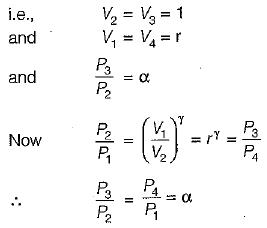Work done - area of the P-V'diagram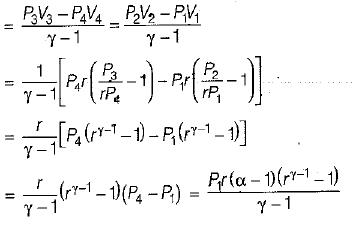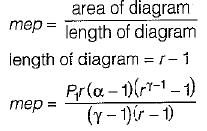Hence mean effective pressure is directly proportional to pressure ratio (α) i.e., P1/P2

QUESTION: 2

### For a given compression ratio the work output of Otto cycle is

Solution:

As compression ratio increases, the thermal efficiency of cycle increases and for the same heat input workout put increases.

QUESTION: 3

### For a given value of compression ratio, efficiency of Otto cycle

Solution:

Air standard efficiency of Otto cycle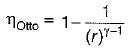Hence thermal efficiency or air standard efficiency of Otto cycle is proportional to the compression ratio.

QUESTION: 4

For an Otto cycle, the increase in compression ratio leads to

Solution:

Thermale fficiency of an Ottocycle is proportional to the compression ratio only and increase in compression leads the higher thermal efficiency and for same heat input higher workout put. Hence increase in mean effective pressure.

QUESTION: 5

In an air-standard diesel cycle; r is the compression ratio, p is the fuel cut-off ratio and γ is the adiabatic inde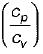Its air standard efficiency is given by

Solution: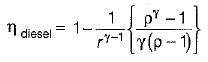QUESTION: 6

In air standard" Diesel cycle at fixed compression ratio and fixed γ,

Solution:

With increase in heat addition for same power output efficiency of diesel engine decrease but with increase in cutoff ratio thermal efficiency decreases.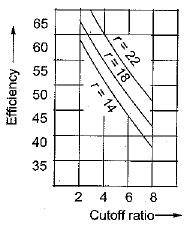QUESTION: 7

For dual combustion cycle for fixed value of heat addition and compression ratio

Solution:
QUESTION: 8

For the same compression ratio and heat addition

Solution: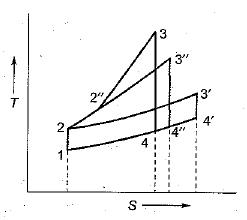Otto cycle => 1-2-3-4
Diesel cycle =>1-2-3'-4'
Dual cycle => 1-2-2"-3"-4"-1

All the cycles starts from the same initial state 1 and the air is reversible adiabatically compresed to state 2, as the compression ratio is same. For the same compression ratio and heat input, minimum heat is rejected in the Otto cycle arid the maximum heat is rejected in the Diesel cycle. Consequently, the Otto cycle has largest work area hence highest efficiency, the Diesel cycle has least efficiency, the Dual cycle having efficiency between these two.
Hence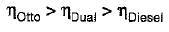QUESTION: 9

When the engines are built to withstand the same thermal and mechanical stresse

Solution:
QUESTION: 10

For the same compression ratio and heat rejection

Solution: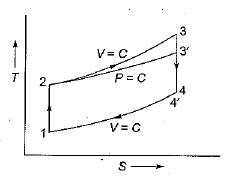For the same compression ratio and heat rejection, heat supplied is more in Otto cycle and hence it will be more efficient than Diesel and Dual will be in between these two.
Hence